# ICSE Class 9 Physics Sample Question Paper 2 with Answers

## ICSE Class 9 Physics Sample Question Paper 2 with Answers

Section -1
(Attempt all questions from this section)

Question 1.
(a) Define the light year. Express it in metre.
(b) A student measures the length of a line with a (ordinary) meter scale and quotes his result as 15.78 cm. Is this result acceptable?
(c) Name the two factors on which the time period of a simple pendulum depends.
(d) Fill in the blanks :
(i) The S.I. unit of pressure is …………………..
(ii) The backlash error can be there in a …………………..
(e) The time periods of two simple pendulums are in the ratio 2:1. What will be the corresponding ratio of their lengths?
(a) A light year is defined as the distance travelled by light, in vacuum, in a time span of one
1 light year = 9.46 x 1015 m

(b) No. the meter scale can measure lengths only up to 0.1 cm, i.e., only up to the first decimal place. Hence, he is not justified in quoting his result up to the second decimal place.

(c) The time period of a simple pendulum depends upon (1) its effective length (l), and (2) the acceleration due to gravity (g) at the place where the pendulum is oscillating.

(d) (i) Pascal (Pa)
(ii) Screw gauge.

(e) For a simple pendulum, we have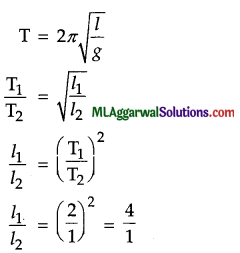Thus, the lengths of the two pendulums are in the ratio 4:1.Question 2.
(a) Draw a diagram to illustrate the motion of a body whose speed remains constant but the velocity continuously changes.
(b) Fill in the blanks :
(i) The acceleration of a body, in uniform motion, equals to ………………..
(ii) A body is said to have a ……………….. when its final velocity is less than its initial velocity.
(c) A body (initially at rest), while going down an inclined plane, has an acceleration of 2 cm/s2. What will be its velocity 5 seconds after the start ?
(d) The displacement-time graph for a particle is as shown in the figure. Is its motion uniform or non-uniform ?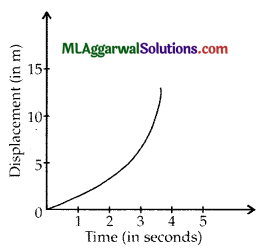(e) A train, moving with a velocity of 20 ms-1, comes to rest in 5 seconds when the brakes are applied. What is its retardation ?
(a) When a body moves with a uniform speed in a circular path, its speed remains constant but its direction of motion (and hence its velocity) continually changes.(b) (i) Zero.
(ii) Retardation

(c) We know,
v = u + at
Given : u = 0, a = 2 cm/s2, t = 5 s
v = 0 + 2 x 5 = 10 cm/s = 0.1 m/s

(d) The motion of the particle is non-uniform. This is because the displacement-time graph is a (parabolic) curve. It is not a straight line as it should be for a uniform motion.(e) Given: u = 20 ms-1, v = 0, t = 5 s
υ = u + at
0 = 20 + a x 5, where ‘a’ is the acceleration
Thus $$a=\left(\frac{0-25}{5}\right)=-4 \mathrm{~ms}^{-2}$$
The retardation is 4 ms-2.

Question 3.
(a) A pile of books is kept on a cardboard sheet on a table. If the cardboard sheet is suddenly withdrawn, what happens to the pile, and why?
(b) Tractors usually have very wide rear wheels. Why ?
(c) When balloons are filled with hydrogen, they are seen rising towards the ceiling. However, when they are filled through the mouth, they sink to the floor. Explain why this happens ?
(d) Why does an object appear lighter, when suspended in a liquid ?
(e) Consider an iceberg floating in fresh water. Find the fraction of volume of the iceberg which is below the water surface. (Given : density of ice = 917 kg m-3, density of fresh water = 1000 kg m-3.)
(a) The pile of books will remain intact. This is because of “inertia of rest”. When the cardboard sheet is suddenly withdrawn, the books continue to remain at rest because of their inertia.

(b) Tractors are used in fields where the soil is quite soft. Thus, if they exert a large pressure on the ground, their wheels will sink into the soft soil. However, use of wide rear wheels will decrease the pressure of their weight on the ground. This helps them to move on soft soil without getting stuck.

(c) The balloons experience an upthrust due to the air in the room. The density of hydrogen is considerably lower than that of air. The upthrust on a balloon filled with hydrogen is, therefore, more than its own weight. The balloon is therefore, pushed up and is seen rising towards the ceiling.

The density of air exhaled by the mouth is more than the density of the room air because of the presence of a large amount of carbon dioxide in the exhaled air. The weight of balloon filled through the mouth is more than the upthrust on it. This balloon, therefore, sinks to the ground.-

(d) The object experiences an upthrust, or a buoyant force, when suspended in a liquid. Because of this, the net downward force on it becomes smaller. It, therefore, appears lighter.(e) Let V be the volume of the iceberg, and v’ that of its part below the water surface. We then have,
Weight of iceberg = Upthrust due to displaced water
Or V x 917 x g = υ‘ x 1000 x g
$$\frac{v^{\prime}}{\mathrm{V}}=\frac{917}{1000}=0.917$$

Question 4.
(a) Fossil fuels are energy rich compounds of an element X which were originally made by the plants with the help of sun’s energy.
(i) Name the element X.
(ii) Name another element which is usually found in combination with X in fossil fuels.

(b) State two characteristics which a source of energy should have.
(c) What do we understand by the term ‘optical medium’ ? Give example.
(d) Thunder is heard 8 seconds after a lightning flash. Calculate the distance in which the lightning occured if the mean temperature of the air is 20°C, velocity of sound in air at 20°C is equal to 340 m/s.
(e) Distinguish between heat and temperature.
(a) (i) Carbon
(ii) Hydrogen

(b) A source of energy must have the following characteristics :
(1) It should be capable of providing adequate useful energy.
(2) It should be convenient to use.

(c) Any medium through which light passes is referred as an optical medium. For example, glass, water, air etc.

(d) As the thunder is heard 8 s after the lightning flash, the distance sound travelled in this interval of
time is :
340 x 8 = 2720 m.
The lightning occurred at a distance of 2720 m.(e)

 Heat Temperature Heat is form of energy obtained due to random motion of mole­cules in a substance. Temperature is a quantity which determines the direction of flow of heat on keeping the two bodies at different temperature in contact. The S.I. unit of heat is joule (J). The S.I. unit of temperature is kelvin (K).

Section – II
(Attempt any four questions from this section)

Question 5.
(a) Which instrument would be preferable for measuring lengths to an accuracy of :
(i) 1 mm, (ii) 1 mm, and (iii) 0.01 mm ?

(b) (i) A girl is swinging on a swing in a sitting position. How will the period of the swing be affected if she stands up ?
(ii) The bob of an oscillating pendulum is made of ice. How will the time period change if the ice starts melting ?
(iii) Will a pendulum gain or lose time when taken to a mountain ?

(c) A screw gauge has a pitch of 0.5 mm and there are 100 divisions on its circular head. When it was used to measure the diameter of a wire, the main scale reading was 3 mm and the 45th division of the circular head was seen to be coincident with the reference line. The zero of its circular division was seen to be coincident with the zero of the main scale when the two jaws were put in contact. What is the diameter of the wire ?
(a) In each case, we would need an instrument whose least count equals the desired accuracy. Thus, the instruments needed are :

• a meter scale
• a vernier calliper, and
• a screw gauge.

(b)
(i) As a girl stands up, the centre of gravity of the swing is raised up, i.e.r the length 7′ of the system decreases. Hence, the time period $$\left(\mathrm{T}=2 \pi \sqrt{\frac{l}{g}}\right)$$ decreases.
(ii) The time period will not be affected because it is independent of the mass of the bob.
(iii) When a pendulum is taken to a mountain-top, g will decrease. Thus, $$\mathrm{T}=2 \pi \sqrt{\frac{l}{g}}$$ will U increase, i.e., the pendulum will take more time to complete one oscillation. In other words, it will lose time.

(c) We have, ‘zero error’ in the given screw gauge = nil.
Pitch 0.5
Least count $$=\frac{\text { Pitch }}{100}=\frac{0.5}{100}=0.0005 \mathrm{~cm}$$
∴ Diameter of wire = Main scale reading + Coincident circular scale division x Least count
= 0.3 cm + 45 x 0.0005 cm
= (0.3 + 0.0225) cm = 0.3225 cm.Questions 6.
(a) Mention any three differences between the mass and weight of a body.

(b) Calculate the force exerted by the earth on the moon. Take the mass of the earth Me = 6 x 1024 kg and the mass of the moon Mm = 7.4 x 1022 The distance between the earth and the moon is 3.84 x 105 km. Also, G = 6.7 x 10“11 Nm2/kg2.

(c) The table below shows the distance, in cm, travelled by objects A, B and C during each second :

 Time Distance (in cm) covered each second by A, B and C Object A (cm) Object B (cm) Object C (cm) 1st second 20 20 20 2nd second 20 36 60 3rd second 20 24 100 4th second 20 30 140 5th second 20 48 .180

(i) Select the object which is moving with constant speed. Give reason for your choice.
(ii) Select the object which is moving with a constant acceleration. What is the value of this acceleration ?
(iii) Select the object which is moving with a variable acceleration.
(iv) Find how much distance each of the objects A, B and C cover in 5 seconds.

 Mass Weight 1. The mass is the quantity of matter contained in a body. 2. Mass of a body is a scalar quantity 3. Its S.l. unit is kilogram (kg). 1. Weight is the force with which a body is attracted towards the centre of the earth. 2. Weight of a body is a vector quantity. 3. Its S.J. unit is newton (N).

(b)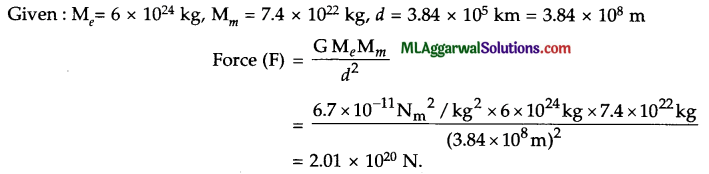The force excerted by the earth on the moon is 2.01 x 1020 N.(c) (i) Object A is moving with a constant speed because it is covering equal distances in each second throughout.
(ii) Object C is moving with a constant acceleration because its speed is increasing at a constant rate each second. The value of this acceleration is 40 cm s-2.
(iii) Object B is moving with a variable acceleration because its speed changes are seen to be irregular.
(iv) Total distance covered by A in 5 seconds = (5 x 20) cm = 100 cm
Total distance covered by B in 5 seconds = (20 + 36 + 24 + 30 + 48) cm
= 158 cm Total distance covered by C in 5 seconds
= (20 + 60 + 100 + 140 + 180) cm
= 500 cm.

Question 7.
(a) A glass jar is kept at a place where the acceleration due to gravity has a value ‘g’ It is filled up to a height ‘h’ with a liquid of density ‘d’. The atmosphere pressure at the place has a value PA.
(i) How much is the pressure at the free surface of the liquid ?
(ii) What would be the total pressure at a point on the base of a jar ?
(iii) Give an expression for the lateral pressure at this depth on the inner side of the jar.

(b) (i) It is said that even a beginner is not likely to sink in the waters of the ‘dead sea’. Why is it so ?
(ii) Define the term ‘relative density of a substance’.

(c) For a given body, it is observed that it weighs 20 gf in air, 18.2 gf in a liquid and 18.0 gf in water. Find :
(i) the relative density of the body, and
(ii) the relative density of the liquid.
(i) Pressure at free surface of the liquid = Atmosphere pressure = PA.
(ii) The total pressure, at the base of the jar equals the sum of atmospheric pressure and the pressure due to liquid column of height 7z’.
∴ Total pressure at the base of the jar = PA + hdg.
(iii) The lateral pressure at this depth on the inner scde of the jar will also be equal to the total pressure, e., lateral pressure = PA + hdg.

(b)(i) The water of the dead sea is extremely salty. Its density is, therefore, much higher than that of fresh water and also more than the average density of the human body. It is because of this large value of density we can say that even a beginner is not likely to sink in the waters of this sea as the sea exerts upthrust more than the body weight.(ii) The relative density of a substance is the ratio of its density to the density of pure water at 4°C, i.e.$$\text { R.D. }=\frac{\text { Density of given substance }}{\text { Density of pure water at } 4^{\circ} \mathrm{C}}$$

(c) Loss of weight of the body in water = (20 – 18) gf = 2 gf
Loss of weight of the body in liquid = (20 – 18.2) gf = 1.8 gf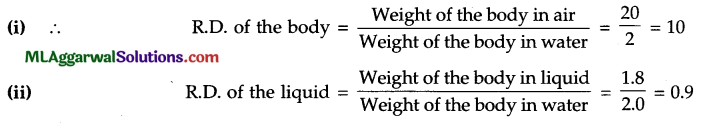Question 8.
(a) An object is placed at a distance of 2 cm from a plane mirror. If the object is moved by 1 cm towards the mirrors, what will be the new distance between the object and its image ?

(b) Fill in the blanks :
(i) When the rays do not actually meet, we get a …………… image.
(ii) Two parallel plane mirrors can be used to get …………. images of an object.
(iii) The image formed by a plane mirror is …………..with respect to the object.

(c) Redraw the following diagram. Complete it by drawing two rays to show the formation of the image of on object AB. What is the size, position and nature of the image formed ?(a) Initial distance between the object and its image = (2 + 2) cm = 4 cm.
Final distance between the object and its image = (1 + 1) cm = 2 cm.(b) (i) Virtual (ii) Infinite (iii) Laterally inverted.

(c) The required rays are as shown. The image formed is :
(1) Smaller in size than the object,
(2) Formed between P and F, i.e., the pole and the focus of the mirror.
(3) Virtual in nature.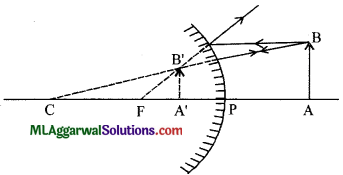Question 9.
(i) Give three points of distinction between the propagation of sound and that of light.
(ii) A longitudinal wave of wavelength 1 cm travels in air with a speed of 330 ms-1. Calcu­late the frequency of the wave. Can this wave be heard by a normal human being ?
(iii) Establish a relation between velocity, frequency and wavelength of a wave.
(a) (1) The speed of light in air is 3 x 108 ms-1 whereas the speed of sound in air is 330 ms-1 at 0°C.
(2) The speed of light decreases in an optically denser medium (speed of light in water is 2.25 x 108 ms-1, in glass is 2.0 x 108 ms-1), while the speed of sound is more in solids, less in liquids and least in gases.
(3) Light can travel in vacuum, but sound cannot travel in vacuum.

(b) Given : λ = 1 cm = 0.01 m,υ = 330 ms
We know,  υ =fλ
∴ $$f=\frac{\lambda}{v}=\frac{330}{0.01}=33000 \mathrm{~Hz}$$
No, this cannot be heard by a normal human being.

(c) Let the velocity of a wave be ‘υ’, time period ‘T’, frequency ‘f’ and wavelength ‘λ’. By the definition of wavelength.
Wavelength λ = Distance travelled by the wave in one time period, i.e., T second
= Wavelength × Time Period
or λ = υ × T
But $$\mathrm{T}=\frac{1}{f}$$
υ = f × λQuestion 10.
(a) Name the following :
(i) Instrument used to control current in an electric circuit
(ii) Instrument used to detect current
(iii) Instrument used to measure current.
(b) State three evidences to show the existence of earth’s magnetic field.
(c) A conductor carries a current of 0.4 A.
(d) Find the amount of charge that will pass through the cross-section of conductor in 16 s.
(e) How many electrons will flow in this time interval if charge on one electron is 1.6 x 10-19 C ?
(a) (i) Rheostat
(ii) Galvanometer
(iii) Ammeter.

(b) The existance of the earth’s magnetic field is based on the following facts :
(1) An iron piece buried inside the earth becomes a magnet.
(2) Neutral points are obtained when the lines of force of a bar magnet are drawn.
(3) A freely suspended needle always points in one direction (north-south direction).

(c) Given : I = 0.4 A, t = 16 s
(i) Charge = Current x Time
or Q = I x t = 0.4 x 16 = 6.4 C
(ii) If V electrons flow and ‘e is the charge on one electron, then total charge passed
Q = ne
$$n=\frac{\mathrm{Q}}{e}=\frac{6.4}{1.6 \times 10^{-19}}=4 \times 10^{19}$$